Python Functions
• 10 Sep 2020
• 1 Minute to read
• Contributors• Dark
Light

# Python Functions

• Dark
Light

## Animated Walkthrough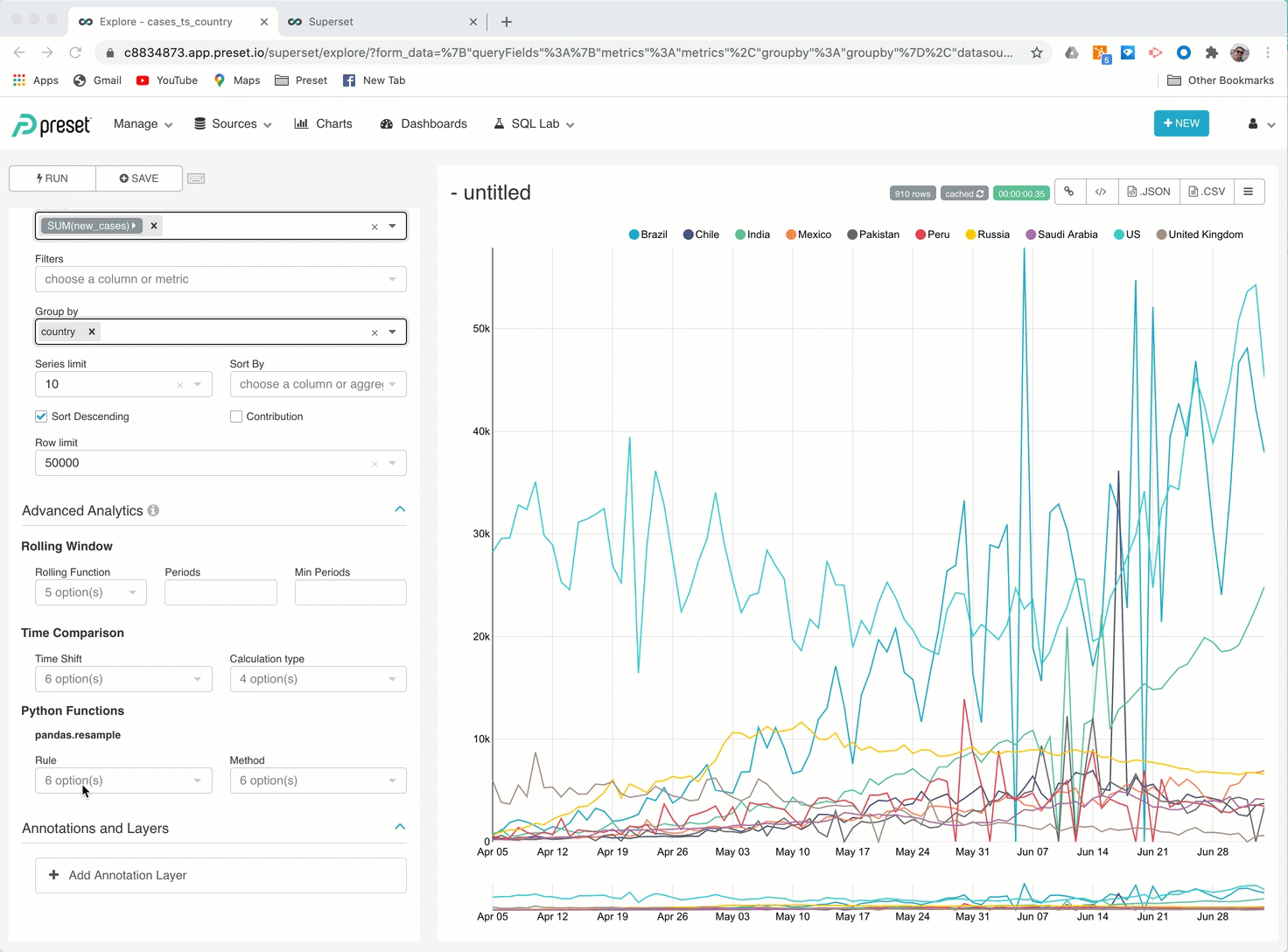## Python Functions

Data fetched from the database are placed in a pandas DataFrame to perform analytics functions.

Python functions are useful in these scenarios:

• Databases that do not support advanced analytics.
• When there is missing data for some time periods — Python functions enable you to perform gap filling with values depending on the function selected.
• Group data based on Period and show values based on Method.

## Rule

Rule is to set up additional grouping to represent the data. Data in this group will apply formulas in Method and this will be drawn in the chart. There 10 default options, but you can insert new options as needed provided that you follow the syntax of assigning a letter(s) to rules, such as:

• S = Seconds
• T = Minutes
• H = Hours
• W = Weeks
• D = Days
• M = Months
• AS = Years

## Method

Function to apply on grouped data based on Rule as defined:

• asfreq: Returns current data.
• bfill: If data is missing, it will do a gap filling with the previous grouping value.
• ffill : If data is missing, it will do a gap filling with the next grouping value.
• median: Half point value where 50% of the values are smaller and 50% are bigger than the value for the period defined in the rule starting from this point to the next data point.
• mean: Average value or values for the period defined in the rule starting from this point to the next data point.
• sum: Sum of values in the period specified in Rule.

## Example of Python Functions

### Chart Without any Python Function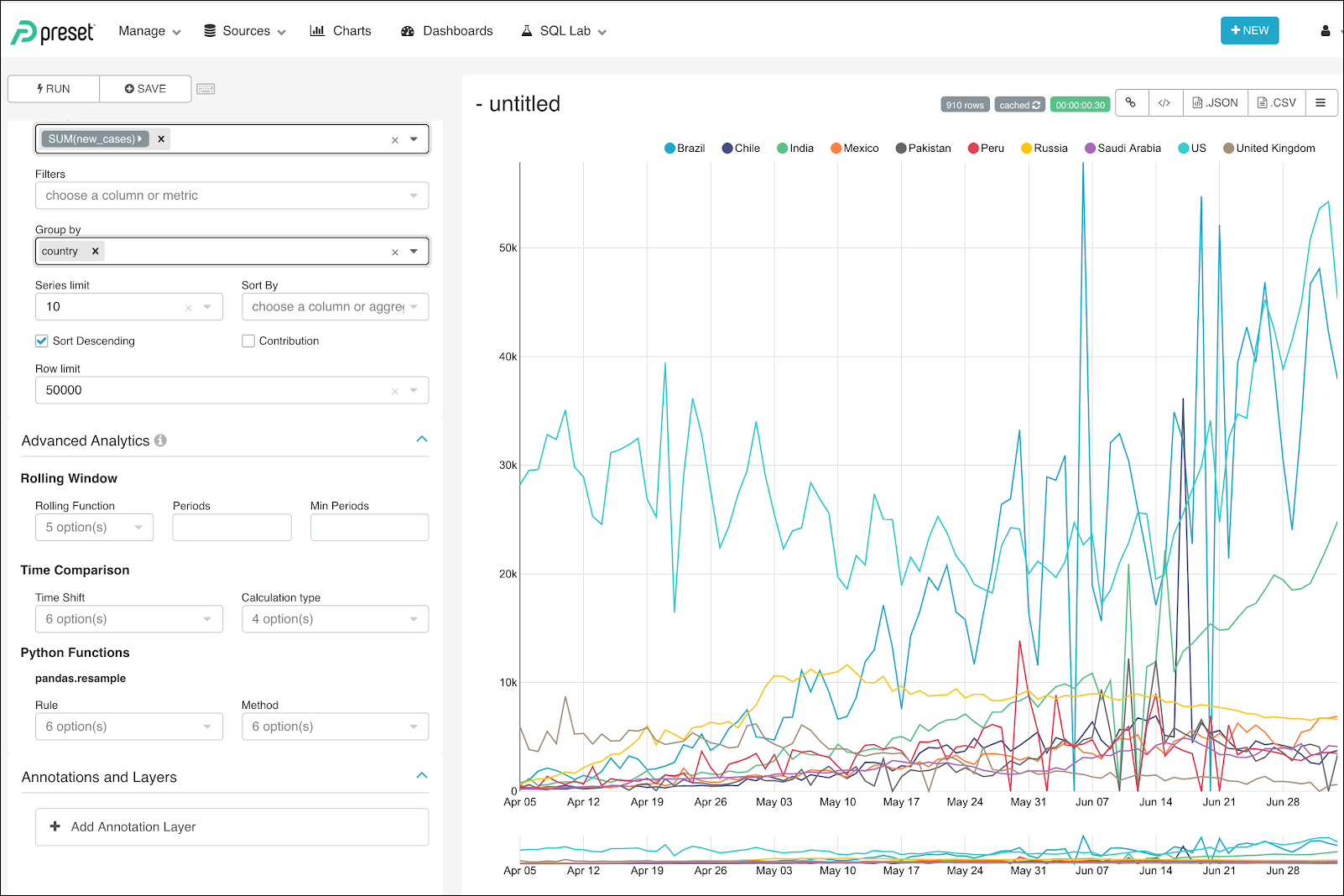### Rule 7D - Method asfreq

In this example, we can see actual values every 7 days, but not showing values between those 7 days.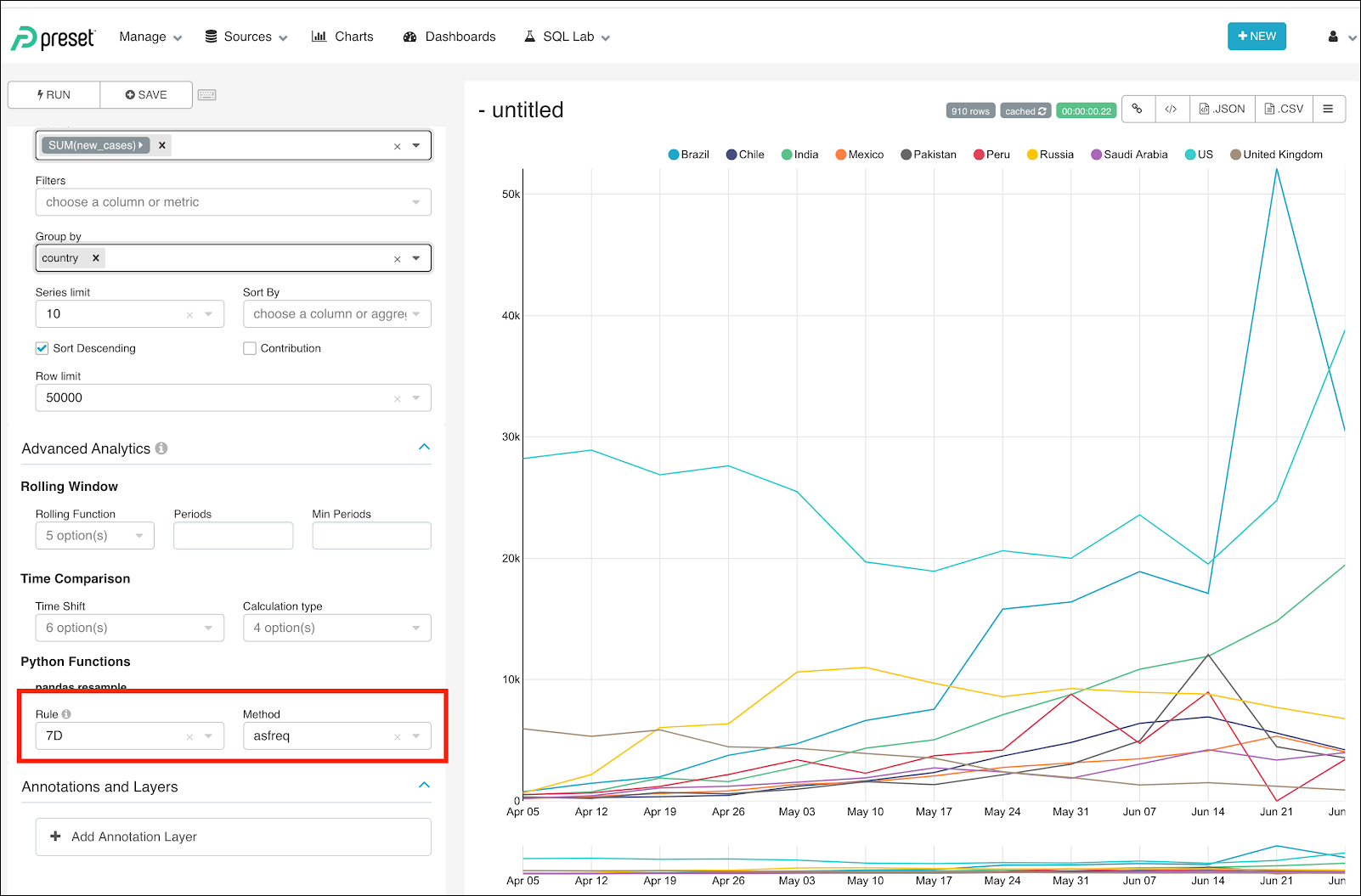### Rule 7D - Method median

Shows a value every 7 days, with each value representing the Median of the data for those 7 days.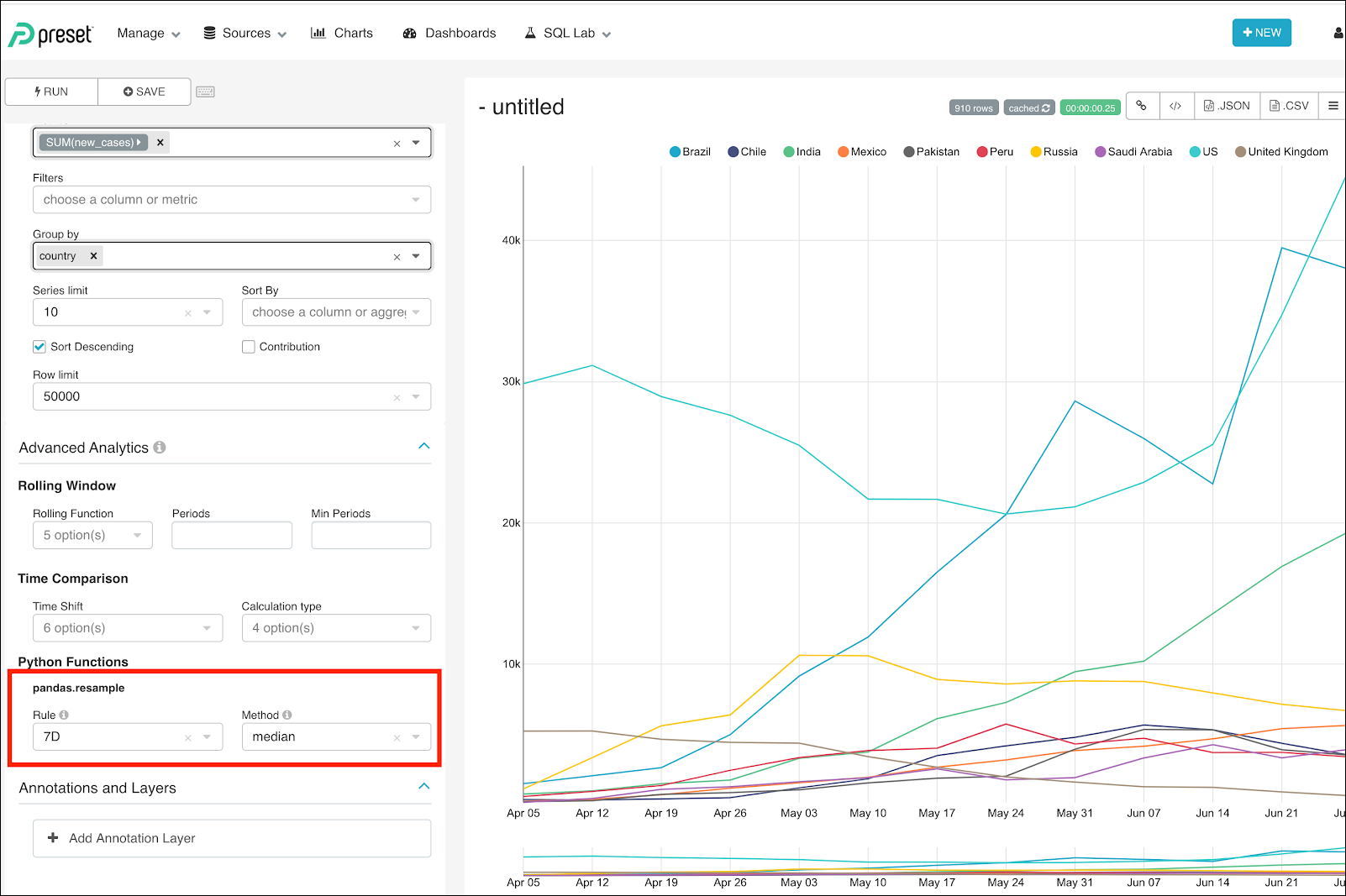### Rule 10D - Method sum

Shows the sum of values in the period of 10 days.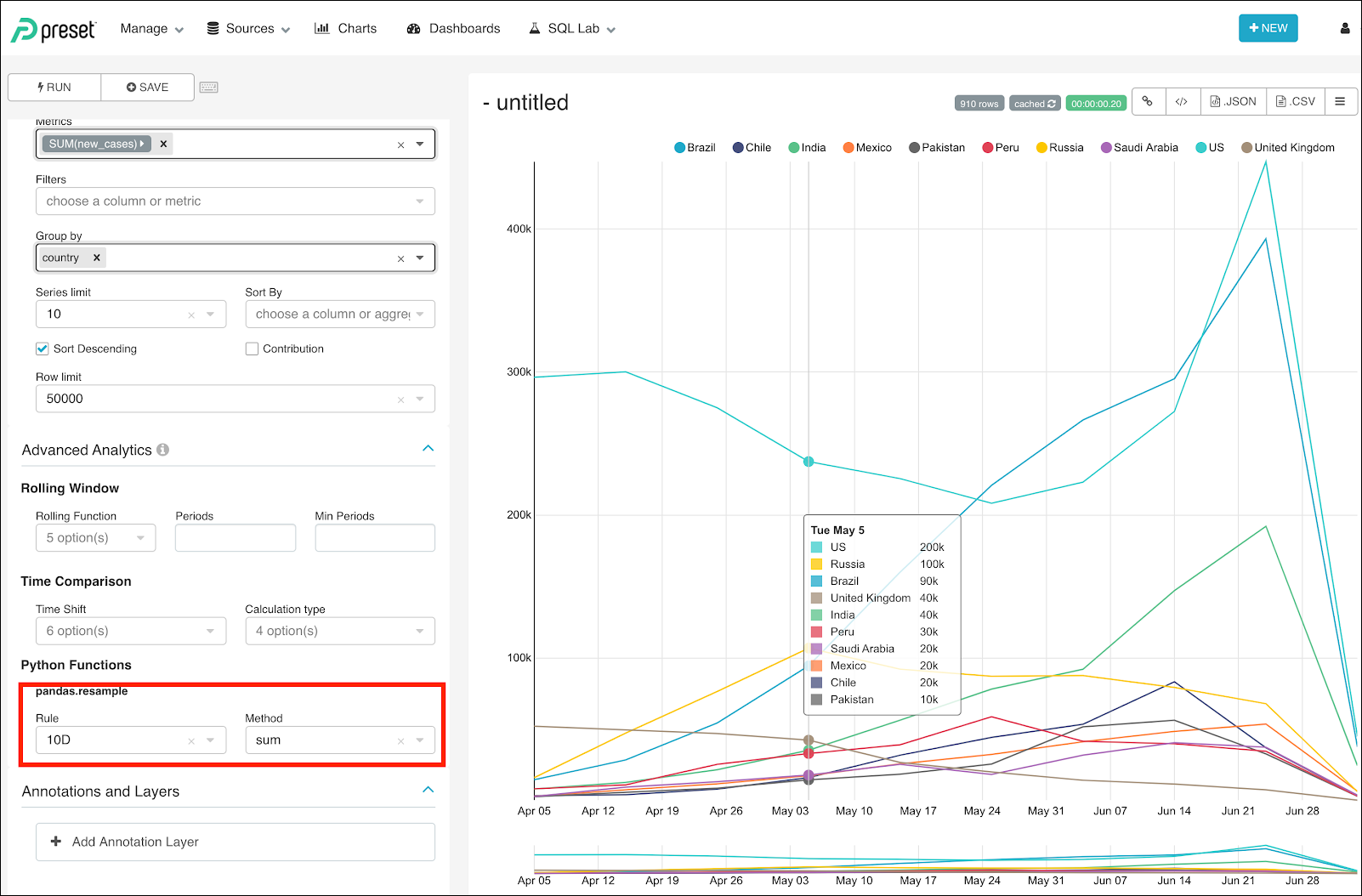When doing sums, consider the last period. If there are not enough dates to complete a RULE, then it can appear to be a decrease in data, but this is because the period has less days than the complete periods.